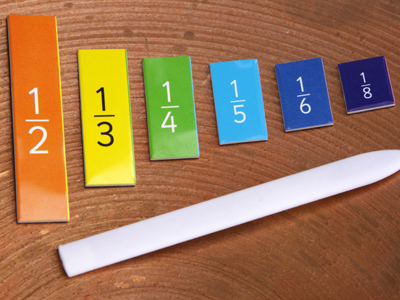Test your fractions skills in this quiz.

# Fractions (Difficult)

All fractions have a numerator and a denominator. Before you do this quiz, do all the other 11-plus Maths quizzes on fractions.

By the time you come to play this quiz, you should have a good grasp of fractions. Remember the best way to learn any new subject is to keep practising every day until you are confident you know what you are doing.

As always, take your time in this quiz. Read the questions carefully - even read them aloud to help - and look at all four answers before choosing. If you get it wrong (or even if you get it right), make sure you read the comment that pops up after the question has been answered. These comments will help you understand how the calculation is worked out.

Good luck!

1.
What is 548 as an improper fraction in its simplest form?
448
112
224
52
To convert a mixed fraction to an improper fraction, follow these steps: 1. Multiply the whole number part by the denominator. 2. Add this result to the numerator. 3. Write the fraction with step 2 in the numerator and keep the original denominator. STEP 1: 5 × 8 = 40. STEP 2: 40 + 4 = 44. STEP 3: 448. Divide the numerator and denominator by 4 to reduce the fraction to its simplest form: 112
2.
If 1730 of the class voted against extra homework, how many pupils in the class voted in favour of extra homework?
17 pupils
13 pupils
1330 of the class
235
1330 of the class voted in favour of extra homework BUT fractions alone DO NOT tell you the actual number of people or things involved. If you had been told that there were 30 pupils in the class, then choice 2 would have been the best answer. Always be on the lookout for the BEST answer
3.
An eighth of an eighth is the same as what fraction of a fourth?
12
116
18
34
The word OF means multiply: 18 × 18 = 164. You now have to find a fraction which multiplied with 14 gives 164. Well, 4 × 16 = 64, so the fraction (in its simplest form) must be 116
4.
What is 247 as a mixed fraction in its simplest form?
2107
3724
337
737
To convert an improper fraction to a mixed fraction, follow these steps: 1. Divide the numerator by the denominator. 2. Note the whole number remainder. 3. Write the number from step 1 as the whole number in front of the fractional part AND write the fractional part with the remainder in the numerator and keep the original denominator. STEP 1: 24 ÷ 7 = 3. STEP 2: Remainder 3. STEP 3: 337
5.
What is four-fifths of five-sixteenths in its simplest form?
54
2080
45
14
The word OF means multiply: 45 × 516 = 14. You multiply the numbers in the denominator together, and you multiply the numbers in the numerator together to form a single fraction. This fraction can then be reduced to its simplest form: sometimes you can reduce the fractions before you multiply them together
6.
There are 360° in a circle. If you walk round 58 of the circumference of a circle, how many degrees will you have walked around?
225°
576°
125°
252°
All you have to do is find 58 of 360°: 58 × 360° = (5 × 360°) ÷ 8 = 1,800° ÷ 8 = 225°. Do the brackets first
7.
How many 615 are there in 45?
4
24
2
3
615 can be reduced to 25 (divide the numerator and the denominator by 3). Now you can see that 45 is twice as much as 25
8.
What is 184 as a mixed fraction in its simplest form?
412
326
413
424
To convert an improper fraction to a mixed fraction, follow these steps: 1. Divide the numerator by the denominator. 2. Note the whole number remainder. 3. Write the number from step 1 as the whole number in front of the fractional part AND write the fractional part with the remainder in the numerator and keep the original denominator. STEP 1: 18 ÷ 4 = 4. STEP 2: Remainder 2. STEP 3: 424. Divide the numerator and denominator by 2 to reduce the fraction to its simplest form: 412
9.
What is 46 + 83 + 515 expressed as a mixed fraction?
332
223
346
323
46 + 83 + 515 = 23 + 83 + 13 = 113 = 323. Reduce the fractions to their simplest forms, then add them. If the denominators are the same, you can add/subtract the fractions by simply adding/subtracting their numerators - it really is as easy as that! Finally, write the improper fraction as a mixed fraction
10.
What is 968 as an improper fraction in its simplest form?
728
274
392
394
To convert a mixed fraction to an improper fraction, follow these steps: 1. Multiply the whole number part by the denominator. 2. Add this result to the numerator. 3. Write the fraction with step 2 in the numerator and keep the original denominator. STEP 1: 9 × 8 = 72. STEP 2: 72 + 6 = 78. STEP 3: 788. Divide the numerator and denominator by 2 to reduce the fraction to its simplest form: 394
Author:  Frank Evans